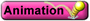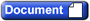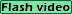About C.O.R.E.
CHAPTERS1-Introduction2-Phys. & Chem. Prop.3-Atomic Structure Pt.14-Nuclear5-Moles, Molarity,
Reaction Types6-Stoichiometry7-Gas Laws8-Atomic Structure Pt.29-Bonding & Geometry10-Intermolecular Forces11-Thermochemistry12-Colligative Properties13-Thermodynamics14-Gas Phase, Solubility,
Complex Ion Equilibria15-Kinetics16-Acid-Base Equilibria17-Electrochemistry

 Chapter One - IntroductionAnimations and DocumentsVolume Unit Conversions This animation visually compares the relationship between a cubic meter, cubic decimeter, cubic centimeter, and cubic milllimeter.CuCl2 and Iron Nail Lab Document This is the document for the reaction of CuCl2 and an iron nail lab. Videos Significant Figures This video introduces the concept of significant figures in measurements. optional versionIntroduction Chapter 1 This video is the introduction to Chapter 1 of the web course. optional versionCuCl2 and Iron Nail Lab Part 1 This video shows the setup of the long term reaction of CuCl2 and an iron nail. (Document) optional version